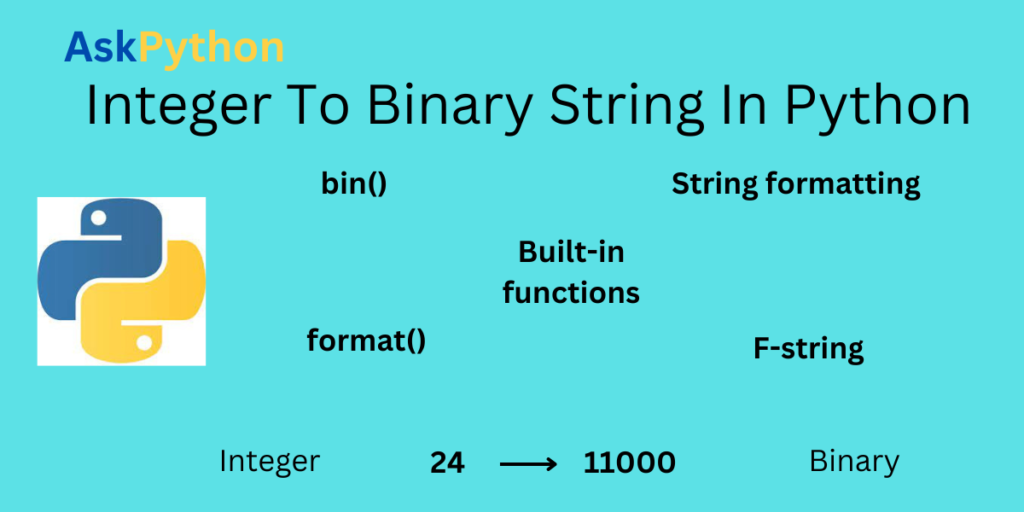# Integer to Binary String in PythonHello readers, in every programming language, we use different data types to do easy and complex operations. Data types are the building blocks of every programming language. The integer data type is one of them. In this article, we will learn how to convert an integer to a binary string in python. This conversion is used while solving complex coding questions because the computer understands the binary language only.

We can use different functions like a `bin()`, `format()`, and other f-strings and string formatting techniques for integer to binary string conversion.

## What is an integer to binary string conversion?

when we write a value of integer data type in the code, it is always in the form of base 10. simple integer values combine ten numbers ranging from 0 to 9. But, computer-understandable language is binary language. binary language is represented in the form of base 2. Binary language is nothing but the combination of 0’s and 1’s. Now let’s learn about the techniques and functions of this conversion.

## bin() function for integer to binary string conversion

`bin()` function is a built-in function in python that is used to convert integer numbers into the binary string format. In This very easy way of conversion, only we have to pass the integer value to the `bin() `function. The output of this function is a binary string representation of the integer number along with the 0b prefix.

```bin(integer)
```

### Example 1: conversion of int to binary string using bin() function for positive integer

In this example 1, we will write 3-line code to convert the positive integer number into a binary string.

```posinteger = 777
binarystring = bin(posinteger)
print(binarystring)
```

### Example 2: conversion of int to binary string using bin() function for negative integer

We will execute the same code of example 1 again but with the negative integer.

```neginteger = -777
binarystring = bin(neginteger)
print(binarystring)
```

### Output:

In the output of example 2, you can understand that this is a binary string conversion of a negative integer. The output of example 2 represents a binary string with a prefix. So, it becomes easy to know the type of integer is positive or negative using the `bin()` function in python.

## format() function for integer to binary string conversion

Another built-in function in the python language for integer to binary string conversion is the `format()` function. `format()` function takes an integer value as an argument and converts it into the specified format. For example, using the `format()` function, we can change the integer into the binary, octal, or hexadecimal string format.

```format(value, value specifier)
```

value specifier is a type in which we want to convert our integer data. value is the integer data. Now let’s see the example to understand the process of `format()` function for integer to binary string conversion.

### Example 3: conversion of int to binary string using format() function

```posinteger = 24
neginteger = -24

pos_binary = format(posinteger,'b')
neg_binary = format(neginteger,'b')

print(pos_binary)
print(neg_binary)
```

### Output:

In example 3 we have implemented the conversion using the format() function for positive and negative integers.

## string formatting for integer to binary string conversion

when we use the `bin()` function for integer to binary string conversion in python, the output always prints with the 0b prefix. This string formatting technique can remove this prefix in python.

### Example 4: convert int to binary string using string formatting technique

```posinteger = 24
neginteger = -24

pos_binary = '{0:b}'.format(posinteger)
neg_binary = '{0:b}'.format(neginteger)

print(f'{pos_binary=}')
print(f'{neg_binary=}')

```

### Output

The output of example 4 shows that the binary string is printed without the 0b prefix.

## f-string in python for integer to binary string conversion

This f-string formatting is similar to traditional string formatting in python. We have to add ‘f’ or ‘F’ prefix in front of the {}. Now let’s implement the f-string formatting on both positive and negative integer to see the results.

### Example 5: f-string formatting for integer to binary string conversion

```posinteger = 7
neginteger = -7

pos_binary = f'{posinteger:b}'
neg_binary = f'{neginteger:b}'

print(f'{pos_binary=}')
print(f'{neg_binary=}')
```

## Conversion of integer to binary string without using built-in functions

We can also convert an integer into binary string format using python’s built-in function or string formatting techniques. For this, we need first to create a function that can convert the integer into a binary string and then pass the integer value to that function. now let’s see how to implement this conversion.

## Example 6: integer to binary string without using built-in function

```def IntegerToBinary(num):

if num >= 1:
IntegerToBinary(num // 2)
print(num % 2, end = '')

if __name__ == '__main__':

Integer_val = 7

IntegerToBinary(Integer_val)
```